# E. FEYNMAN Diagrams

The WICK theorem allows us to evaluate the exact GREEN's functions as a perturbation expansion involving expressions of free GREEN's functions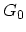and the perturbation potential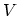(see Section 3.4.1). This expression can be analyzed directly in coordinate or momentum space, in time or energy domain. FEYNMAN introduced the idea of representing different contributions obtained from the WICK decomposition by drawings. These drawings, called diagrams, are very useful for providing an insight into the physical process which these terms represent. The FEYNMAN diagrams provide an illustrative way to solve the many-particle problems and the perturbation expansion of the GREEN's functions.

A diagram dictionary for electrons, which are FERMIons, and phonons, which are Bosons, are shown in Table E. Diagrams for electrons are in coordinate-time space, while phonon diagrams are in momentum-energy space. As described in Section 3.1.1, the GREEN's function can be interpreted as the creation of a particle atin space-time, the propagation of the corresponding perturbation to the point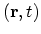in space-time, where the particle is annihilated. Hence, the full GREEN's function is represented by a double line joining these two points. The free GREEN's function is characterized by a single line.

The COULOMB potential is represented by a wavy line with two inputs and outputs which can be coupled together to describe a self-interaction. The COULOMB interaction is assumed to be instantaneous. It is convenient to consider the inter-particle potential as a static instantaneous potential proportional to a delta function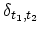.

Intermediate variables describe events taking place between the two space-time arguments of the GREEN's function, but without any constraints for exact time or place. The overall amplitude involves an integration over these variables. Each time a FERMIon loop appears, the perturbation expression corresponding to this FEYNMAN diagram must be multiplied by a factor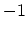.

Electrons can also interact with phonons. For phonons it is more convenient to work in the momentum-energy rather than in the space-time domain. Diagrams concerning a free phonon GREEN's functions and the interaction between electrons and phonons are also shown in Table E. The factor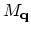refers to the electron-phonon interaction matrix elements.

Table E.1: Feynman Diagrams for electrons (FERMIons) and phonons (Bosons).
 Expression Description Diagram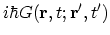Full GREEN's function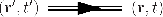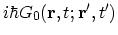Free GREEN's function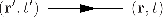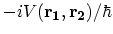COULOMB interaction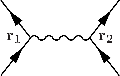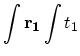Intermediate variable(s)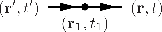FactorAny FERMIon loop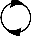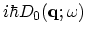Free phonons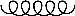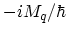Electron-phonon interaction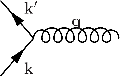M. Pourfath: Numerical Study of Quantum Transport in Carbon Nanotube-Based Transistors